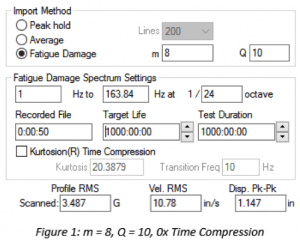# VN0017 - m and Q Values for FDS

## Abstract:Define the effects of m and Q when used for Fatigue Damage Spectrum (FDS) generation, time compression, and combining waveforms.

### Question

How do the m and Q values effect the outcomes of FDS generation, and test compression? What values of m and Q can be used, as a starting point, for test development of a mixed composition component?

For the analysis of a time history file when generating the FDS. A low Q value spreads ‘damage’ out amongst neighboring frequencies, a high Q concentrates ‘damage’. A good starting point to use, if the Q value of a product is not known, is 10. A Q of 10 outputs an FDS, and thus a PSD with reasonable filtering and frequency response. To be more precise, complete a sine sweep of a product and use the Q value of the primary resonance found.

Second, the ‘m’ value is an exponent in the FDS equation, which is why it plays a much larger role than Q, which is a divisor in the FDS equation (Henderson & Piersol, 1995). MIL-STD-810G provides some direction for defining an initial value for m. This is helpful for cases when the S-N curve or m value of a product is not known.

The value of ‘m’ is generally some proportion of the slope of the S-N curve, known as the fatigue strength exponent and designated as ‘b’. Typical values of ‘m’ are 80% of ‘b’ for random waveshapes, and 60% of ‘b’ for sinusoidal waveshapes. Historically, a value of m=7.5 has been used for random environments, but values between 5 and 8 are commonly used. A value of 6 is commonly used for sinusoidal environments. The cumulative damage is based on the fatigue properties of metals. (Defense, 2008)

The m value has differing effects when considering FDS generation, and using FDS for test compression. That said, a number between 5 and 8 serves as a good starting point, but these effects must be taken into consideration.

The type of data that is being imported must be taken into account. Typically, real world data is nonGaussian. For this non-Gaussian data, a higher m weighs the peaks more heavily than a lower m, meaning the high kurtosis peaks have more of a factor in the generation of the FDS. Adding kurtosis to the PSD generated reducesthe effect of the mdue to the decrease in overall GRMS caused by the addition of higher kurtosis peaks. If the input waveform has a kurtosis of 3, the PSD created is the same regardless of mand Q.

When analyzing non-Gaussian waveforms (k > 3) FDS Generation using a higher m weights higher amplitude events more heavily than lower amplitude events, resulting in a test with higher Acceleration, Velocity, and Displacement making it more conservative in relation to the actual imported time history file. Figure 1, and Figure 2 show the resultant PSD profile values when the same time history file is imported, but the m value is changed.For Test time compression when the input waveform is non-Gaussian (k > 3), the m has the opposite effect. For a higher m the PSD amplitude increases less based on an increase in time reduction. Compare Figure 3 and Figure 4. With m = 8, and 2x time compression there is an approximate 10% increase in the profile GRMS and when m=4, with a 2x time compression the increase is 20%. When using time compression, failure is more likely when using a lower m value.Although the damaging peaks of kurtosis in the original time history file are already accounted for in the generated FDS, adding kurtosis to the resultant PSD can be useful, especially in cases where an amplifier may be able to handle the peaks, but unable to maintain the continuous demand of the PSD. Adding kurtosis lowers the overall GRMS requirement of the test while still maintaining an equivalent damage profile to the original imported time history file.

Overall, if the m and Q values for a product are unknown, an initial recommended starting point would be:

• m = 6 or 8 (sinusoidal or random)
• Q=10

These values create an FDS that is conservative, yet has a reasonable amount of filtering and frequency resolution. As more data is accumulated these values can be adjusted to more accurately estimate the m and Q for a product, even if it is a mixed composition component.

For instructions on determining a specific m and Q value see V-Note #0018.

For instructions to create an FDS Test see V-Note #0019.

## Bibliography:

Defense, D. o. (2008, October 31). Test Method
Standard – Mil-STD-810G. Retrieved
July 20, 2018 from https://www.atec.army.mil/.

Henderson, G. R., & Piersol, A. G. (1995, October).
Fatigue Damge Related Descriptor for
Random Vibration Test Environments.
Sound and Vibration, 20-24.